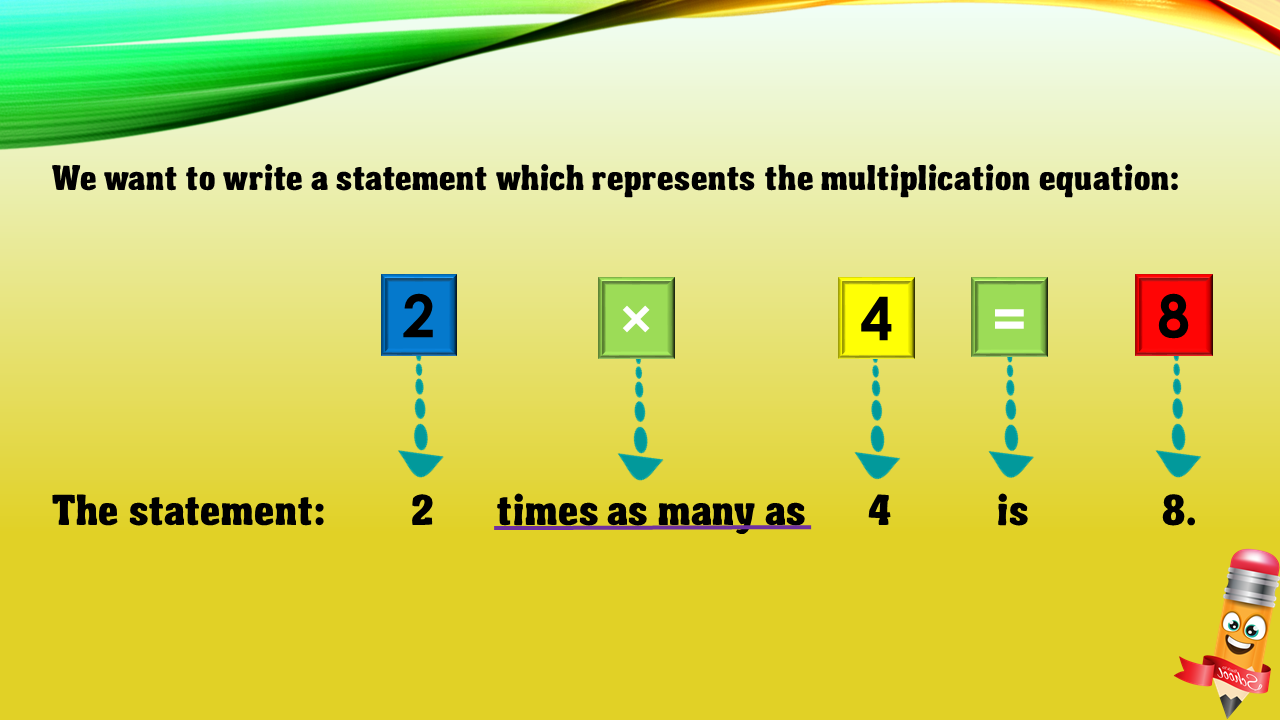1
visibility

Choose one statement which represents the equation:
2 × 4 = 8 ?

• A

2 more than 4 is 8.

• B

4 less than 8 is 2.

• C

2 times as many as 4 is 8.

• D

4 times as many as 8 is 2.